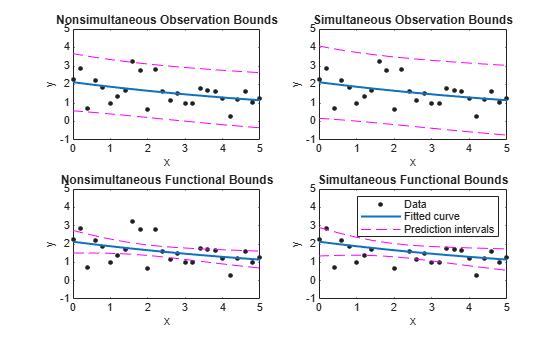Main Content

# predint

Prediction intervals for `cfit` or `sfit` object

## Syntax

``ci = predint(fitresult,x)``
``ci = predint(fitresult,x,level)``
``ci = predint(fitresult,x,level,intopt,simopt)``
``[ci,y] = predint(...)``

## Description

example

````ci = predint(fitresult,x)` returns upper and lower 95% prediction bounds for response values associated with the `cfit` object `fitresult` at the new predictor values specified by the vector `x`. `fitresult` must be an output from the `fit` function to contain the necessary information for `ci`. `ci` is an `n`-by-2 array where `n = length(x)`. The left column of `ci` contains the lower bound for each coefficient; the right column contains the upper bound.```
````ci = predint(fitresult,x,level)` returns prediction bounds with a confidence level specified by `level`. `level` must be between `0` and `1`. The default value of `level` is `0.95`.```
````ci = predint(fitresult,x,level,intopt,simopt)` specifies the type of bounds to compute. Observation bounds are wider than functional bounds because they measure the uncertainty of predicting the fitted curve plus the random variation in the new observation. Non-simultaneous bounds are for individual elements of `x`; simultaneous bounds are for all elements of `x`.```
````[ci,y] = predint(...)` returns the response values `y` predicted by `fitresult` at the predictors in `x`. Note`predint` cannot compute prediction intervals for non-parametric regression methods such as `Interpolant`, `Lowess`, and `Spline`. ```

## Examples

collapse all

Compute and plot observation and functional prediction intervals for a fit to noisy data.

Generate noisy data with an exponential trend.

```x = (0:0.2:5)'; y = 2*exp(-0.2*x) + 0.5*randn(size(x));```

Fit a curve to the data using a single-term exponential.

`fitresult = fit(x,y,'exp1');`

Compute 95% observation and functional prediction intervals, both simultaneous and nonsimultaneous. Non-simultaneous bounds are for individual elements of x; simultaneous bounds are for all elements of x.

```p11 = predint(fitresult,x,0.95,'observation','off'); p12 = predint(fitresult,x,0.95,'observation','on'); p21 = predint(fitresult,x,0.95,'functional','off'); p22 = predint(fitresult,x,0.95,'functional','on');```

Plot the data, fit, and prediction intervals. Observation bounds are wider than functional bounds because they measure the uncertainty of predicting the fitted curve plus the random variation in the new observation.

```subplot(2,2,1) plot(fitresult,x,y), hold on, plot(x,p11,'m--'), xlim([0 5]), ylim([-1 5]) title('Nonsimultaneous Observation Bounds','FontSize',9) legend off subplot(2,2,2) plot(fitresult,x,y), hold on, plot(x,p12,'m--'), xlim([0 5]), ylim([-1 5]) title('Simultaneous Observation Bounds','FontSize',9) legend off subplot(2,2,3) plot(fitresult,x,y), hold on, plot(x,p21,'m--'), xlim([0 5]), ylim([-1 5]) title('Nonsimultaneous Functional Bounds','FontSize',9) legend off subplot(2,2,4) plot(fitresult,x,y), hold on, plot(x,p22,'m--'), xlim([0 5]), ylim([-1 5]) title('Simultaneous Functional Bounds','FontSize',9) legend({'Data','Fitted curve', 'Prediction intervals'},... 'FontSize',8,'Location','northeast')```## Input Arguments

collapse all

Function of which you want to find the prediction intervals, specified as a `cfit` or `sfit` object.

`fitresult` must be an output from the `fit` function to contain the necessary information for `ci`.

Predictor values used to compute the upper and lower prediction bounds of `fitresult`, specified as a vector.

Confidence level of the prediction bounds, specified as a positive scalar between `0` and `1`.

Type of bounds, specified as either:

• `'observation'` — Bounds for a new observation (default)

• `'functional'` — Bounds for the fitted curve

Type of bounds, specified as either:

• `'off'` — Non-simultaneous bounds (default)

• `'on'` — Simultaneous bounds

## Output Arguments

collapse all

Upper and lower prediction bounds, returned as an array of size `n`-by-2, where `n = length(x)`.

Response values predicted by `fitresult` at the predictors in `x`, returned as a vector.

## See Also

### Topics

Introduced in R2013a

## Support

#### Machine Learning Challenges: Choosing the Best Classification Model and Avoiding Overfitting

Download white paper